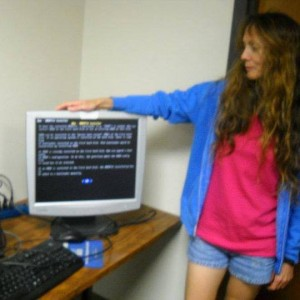Ware Repair closed August 28th, 2020

# The Mathematics Involved in 3D Game Programming - Linear Algebra

### The Mathematics Involved in 3D Game Programming - Linear Algebra

This is a continuation of blogs encompassing 3D game programming information, primarily the necessary mathematics.

See these other blogs first, if you want to be more familiar with the material discussed in this blog:

The Mathematics Involved in 3D Game Programming

The Mathematics Involved in 3D Game Programming - Vector Algebra

And also, these two blogs, which explain how I found WebGL to be a much better 3D programming and learning tool, particularly a certain book I've been following to date (WebGL may be the BEST introduction to 3D programming - Part 1 gives details about this), should be read beforehand likewise:

WebGL and HTML5 Canvas - both provide a good introduction to the fundamentals of game programming and 3D programming

WebGL may be the BEST introduction to 3D programming - Part 1

So, back to the current blog...

In my journey to learn more about the mathematics in 3D programming, I have found that probably the main mathematics discipline involved in 3D programming is linear algebra.

Linear algebra encompasses vector algebra disciplines, as well as vector spaces and matrix arithmetic, all of which are needed in 3D programming. Here is a list from my favorite mathematics instructor, Paul Dawkins from Lamar University, of the main topics involved in linear algebra, from his PDF book Linear Algebra (note that I didn't include every subheading/topic per chapter, but the main topics):

1. Systems of Equations and Matrices - which includes topics such as solving systems of equations as matrices, matrix arithmetic and other operations, properties of matrix arithmetic and the transpose, and inverse matrices.
2. Determinants - which includes an introduction to the determinant function, properties of determinants, and row reduction to compute determinants larger than a 2x2 matrix.
3. Euclidean n-Space - gives explanations of vectors, the dot product and cross product (which were already covered by me in those two vector algebra blogs), Euclidean n-Space (spacial realms beyond 2D and 3D), and linear transforms.
4. Vector Spaces - details about vector spaces, span, linear independence, basis and dimension, fundamental subspaces, inner product spaces, orthonormal bases, and orthogonal matrices.
5. Eigenvalues and Eigenvectors - includes explanations of eigenvalues and eigenvectors, and diagonalization.

Now, why, you may ask, did linear algebra come into play with 3D programming?

In the WebGL book I highly recommended in a previous blog, WebGL Beginner's Guide, by Diego Cantor and Brandon Jones the authors imply that understanding linear algebra operations are necessary to master 3D programming.

I would like to present a few images and information from this book, so to give some idea of what is involved.

Systems of Equations and Matrices

Since I have covered most of this chapter, I can give a little more insight here than with the other chapters in this great book.

Matrices can be created from systems of equations that need to be solved. One thing to keep in mind is solutions to systems of equations can have one solution, infinite solutions, or no solutions. Notice the graphs in these cases:Systems of equations can then be set up as either augmented matrices or coefficient matrices (the b stands for the number to the right of the equal sign):Here is an example of a augmented matrix:These two sections from the book describe (on the left side) the form of the matrix once certain calculations are completed and (on the right side) the symbolism for these particular row calculations:Here are examples of row-echelon form and reduced row-echelon form:And here is an example problem showing the work-flow to the solutions:Determinants

A determinant is basically a function f(X) that takes as its input a matrix X, and then computes a real number from this matrix. A determinant has a lot to do with statistical permutations stemming from the matrix's numbers, which Paul Dawkins explains in much more detail in his book.Euclidean n-Space

Euclidean n-Space is dealing with vectors in spaces higher than 2D or 3D. Paul Dawkins gives a bit of an introduction below. Of course, to learn even more, you would want to read the entire chapter and follow along on all the example problems. Also, as I mentioned in the list of chapters in this book, there is a thorough discussion in this chapter in several subsections about vectors and the dot product and cross product, topics I have already covered in the previous 3D gaming blogs.Vector Spaces

Here is a good definition, from wikipedia, of what is a vector space: "A vector space (also called a linear space) is a collection of objects called vectors, which may be added together and multiplied ("scaled") by numbers, called scalars. Scalars are often taken to be real numbers, but there are also vector spaces with scalar multiplication by complex numbersrational numbers, or generally any field." And here is what Paul has in his book:Eigenvalues and Eigenvectors

Again, here are some wikipedia explanations:

"In linear algebra, an eigenvector or characteristic vector of a linear transformation is a non-zero vector that changes by only a scalar factor when that linear transformation is applied to it. More formally, if T is a linear transformation from a vector space V over a field F into itself and v is a vector in V that is not the zero vector, then v is an eigenvector of T if T(v) is a scalar multiple of v. This condition can be written as the equation

{\displaystyle T(\mathbf {v} )=\lambda \mathbf {v} ,}where λ is a scalar in the field F, known as the eigenvaluecharacteristic value, or characteristic root associated with the eigenvector v."

Paul Dawkins' explanation:### Diane Ware

###### Manager - Tech Support

I am co-owner of Ware Repair and enjoy working with technology, web design and development, and embedded programming, especially that which applies to robotics. I also enjoy writing blogs and sci-fi novels on my free time.